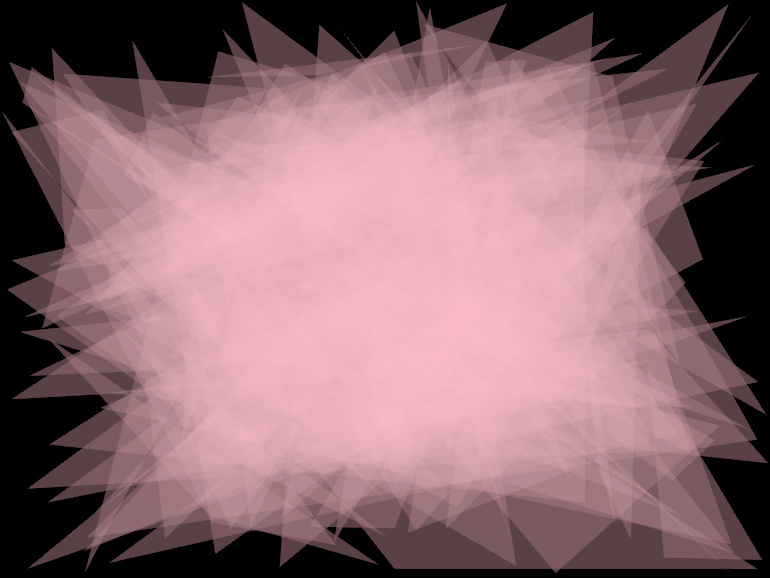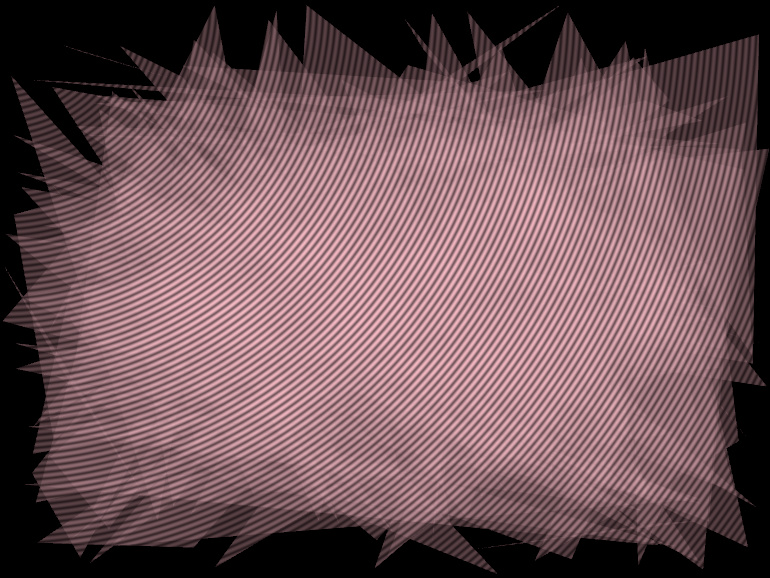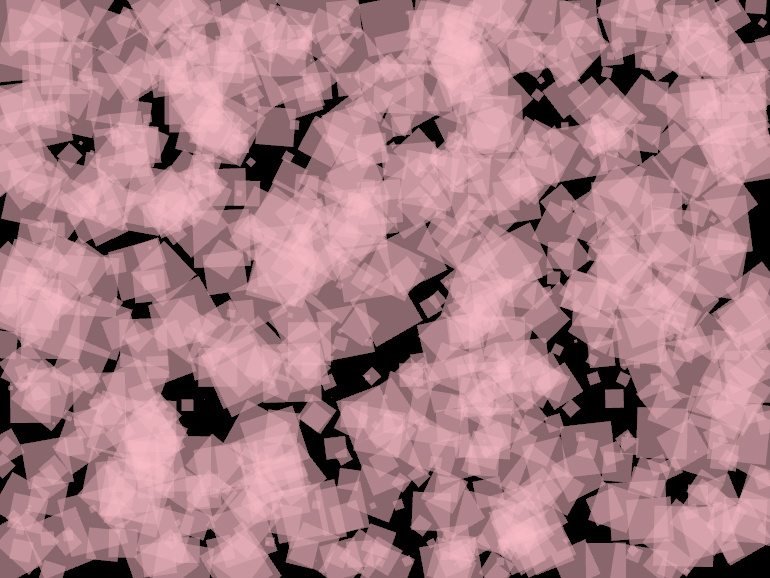# Custom rendering

OPENRNDR is designed with the idea that users should be able to draw beyond the primitives offered by Drawer.

## Vertex buffers

A vertex buffer is a (on the GPU residing) amount of memory in which vertices that describe geometry are stored. A single vertex consists of a number of customizable attributes such as position, normal and color. In OPENRNDR the attributes of a vertex are described using a VertexFormat.

Vertex buffers allow geometry to be prepared and stored in such a way that graphics hardware can draw it directly.

### Declaring a vertex format

A vertex format declaration consists of a list of vertex attributes. Such a declaration is made using the vertex format builder: `vertexFormat {}`

To illustrate the declaration procedure let us give an example of a very simple vertex format. This vertex format consists solely of a three dimensional position attribute:

``````val vf = vertexFormat {
position(3)
}
``````

Listed below are the attributes that can be added to the vertex format.

name type description
`position(dimensions: Int)` `FLOAT32, VECTOR[2,3,4]_FLOAT32` position attribute
`normal(dimensions: Int)` `FLOAT32, VECTOR[2,3,4]_FLOAT32` normal attribute
`textureCoordinate(dimensions: Int)` `FLOAT32, VECTOR[2,3,4]_FLOAT32` texture coordinate attribute
`color(dimensions: Int)` `FLOAT32, VECTOR[2,3,4]_FLOAT32` color attribute
`attribute(name: String, type: VertexElementType, arraySize:Int = 1)` `type` custom attribute

A more complex vertex format declaration would then look like this:

``````val vf = vertexFormat {
position(3)
normal(3)
color(4)
attribute("objectID", VertexElementType.FLOAT32)
}
``````

### Creating a vertex buffer

The vertexBuffer() function is used to create a VertexBuffer instance. For example to create a vertex buffer holding 1000 vertices in our previously defined vertex format `vf` we use the following:

``````val geometry = vertexBuffer(vf, 1000)
``````

### Placing data in the vertex buffer

Now that a vertex format has been defined and a vertex buffer has been created we can place data in the vertex buffer. The data placed in the vertex buffer must closely match the vertex format; any form of mismatch will lead to surprising and/or undefined behaviour.

The `VertexBuffer.put {}` function is the easiest and safest way of placing data in the vertex buffer.

In the following example we create a very simple vertex format that holds just a position attribute. After creation we fill the vertex buffer with random data.

``````val geometry = vertexBuffer(vertexFormat {
position(3)
}, 1000)
geometry.put {
for (i in 0 until 1000) {
write(Vector3(Math.random() - 0.5, Math.random() - 0.5, Math.random() - 0.5))
}
}
``````

### Drawing vertex buffers``````fun main() = application {
program {
val geometry = vertexBuffer(vertexFormat {
position(3)
}, 3 * 100)

geometry.put {
for (i in 0 until geometry.vertexCount) {
write(Vector3(Math.random() * width, Math.random() * height, 0.0))
}
}

extend {
drawer.fill = ColorRGBa.PINK.opacify(0.1)
drawer.vertexBuffer(geometry, DrawPrimitive.TRIANGLES)
}
}
}
``````

Drawing using Drawer.vertexBuffer will be with respect to the set shade style. Using shade styles the appearance of the geometry in the vertex buffer can be fully customized.

The last snippet can be modified to include a simple shading over the geometry``````fun main() = application {
program {
val geometry = vertexBuffer(vertexFormat {
position(3)
}, 3 * 100)

geometry.put {
for (i in 0 until geometry.vertexCount) {
write(Vector3(Math.random() * width, Math.random() * height, 0.00))
}
}

extend {
fragmentTransform = """x_fill.rgb *= vec3(cos(length(v_viewPosition))*0.4+0.6);"""
}
drawer.fill = ColorRGBa.PINK.opacify(0.1)
drawer.vertexBuffer(geometry, DrawPrimitive.TRIANGLES)
}
}
}
``````

## Drawing instances

The same geometry can be drawn more than once in a single draw call.

Per instance attributes can be stored in a vertex buffer. For example a transform per instance can be realized by creating a second vertex buffer which will contain matrix attributes.``````fun main() = application {
configure {
width = 770
height = 578
}
program {
// -- create the vertex buffer
val geometry = vertexBuffer(vertexFormat {
position(3)
}, 4)

// -- fill the vertex buffer with vertices for a unit quad
geometry.put {
write(Vector3(-1.0, -1.0, 0.0))
write(Vector3(-1.0, 1.0, 0.0))
write(Vector3(1.0, -1.0, 0.0))
write(Vector3(1.0, 1.0, 0.0))
}

// -- create the secondary vertex buffer, which will hold transformations
val transforms = vertexBuffer(vertexFormat {
attribute("transform", VertexElementType.MATRIX44_FLOAT32)
}, 1000)

// -- fill the transform buffer
transforms.put {
for (i in 0 until 1000) {
write(transform {
translate(Math.random() * width, Math.random() * height)
rotate(Vector3.UNIT_Z, Math.random() * 360.0)
scale(Math.random() * 30.0)
})
}
}
extend {
drawer.fill = ColorRGBa.PINK.opacify(0.25)